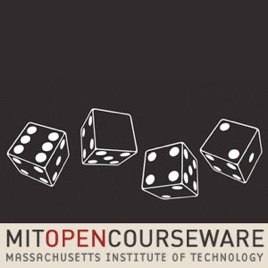44 min# Lecture 1: Introduction and Proofs Mathematics for Computer Science (2010)

• Technology

Introduction to mathematical proofs using axioms and propositions. Covers basics of truth tables and implications, as well as some famous hypotheses and conjectures.

Introduction to mathematical proofs using axioms and propositions. Covers basics of truth tables and implications, as well as some famous hypotheses and conjectures.

44 min